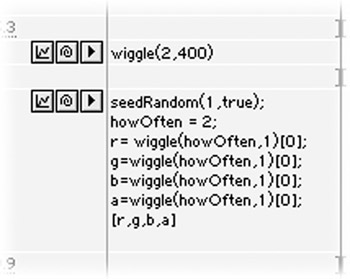# Colorful Expressions II

Expressions think of color as percentage values for the red, green, blue and alpha channels, and the percentages are always numbers between (and including) zero and one. If green is set to .5, that's the same as 50 percent green (or 128 green if you're using the zero to 255 range for RGB values). If you want an expression that wiggles random colors-but you only want random greens hues-try the following:

` seedRandom(1,true); howOften = 2; r=0; g=wiggle(howOften,1); b=0; a=1; [r,g,b,a] `If you want to choose only shades of gray, try this:

` seedRandom(1,true); howOften = 2; r= wiggle(howOften,1); g=wiggle(howOften,1); b=wiggle(howOften,1); a=1; [r,g,b,a] `

You can use this expression to generate interesting random gradients, if you apply the 4 color Gradient effect (in the Render category) to a solid. Add the expression to each of the four Color properties, changing the seedRandom value to a different number each time. For more fun, add a wiggle expression to the color positions (the Point 1 through Point 4 properties).After Effects On the Spot[c] Time-Saving Tips and Shortcuts from the Pros
ISBN: 1578202396
EAN: N/A
Year: 2006
Pages: 447